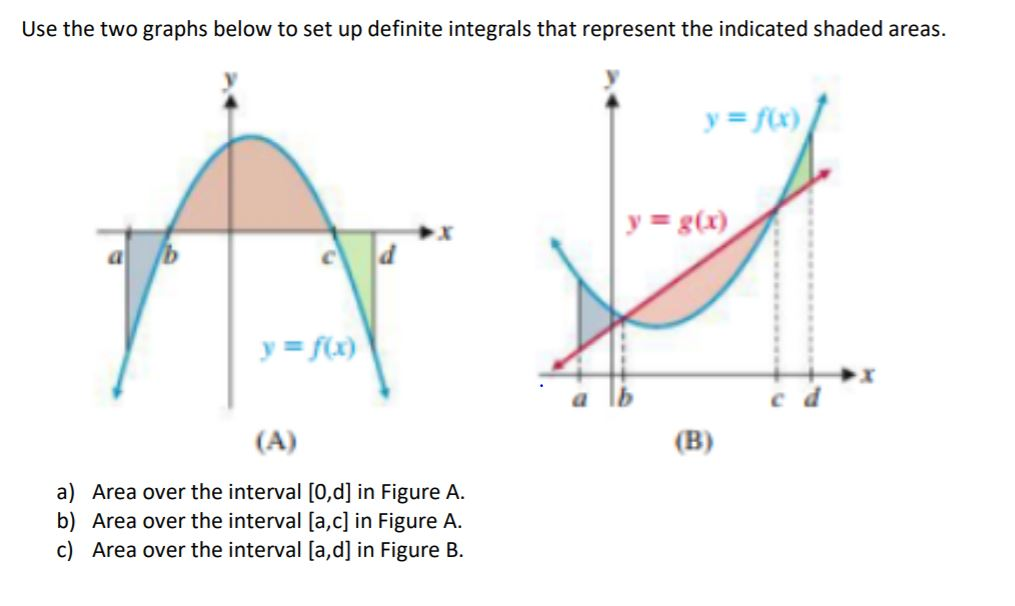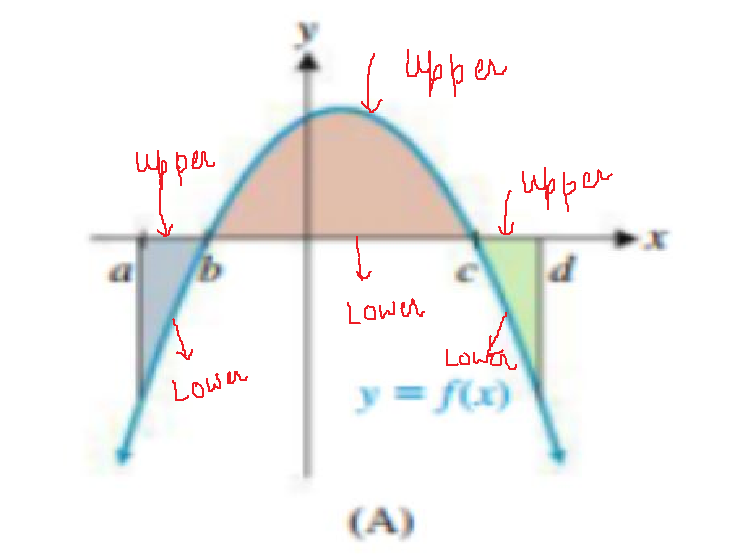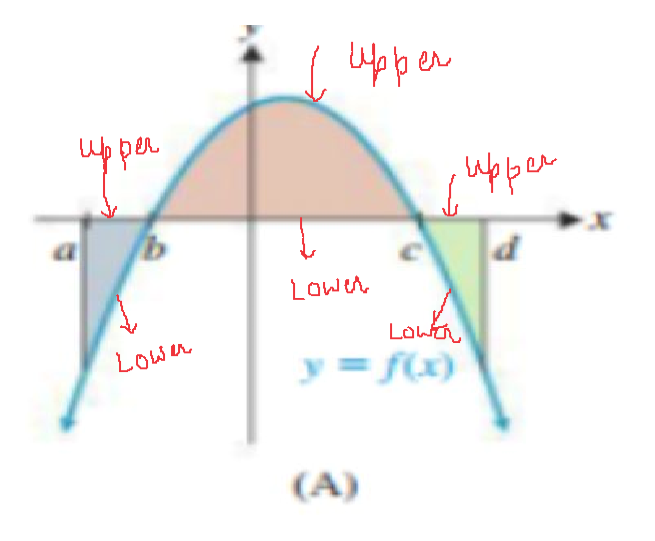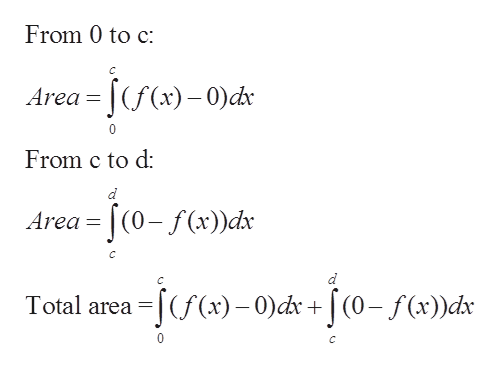Use the two graphs below to set up definite integrals that represent the indicated shaded areas.yfx)y= g(x)yfx)(B)(A)a) Area over the interval [0,d] in Figure A.b) Area over the interval [a,c] in Figure A.c) Area over the interval [a,d] in Figure B

Question

please explain how to solve thishelp_outlineImage TranscriptioncloseUse the two graphs below to set up definite integrals that represent the indicated shaded areas. yfx) y= g(x) yfx) (B) (A) a) Area over the interval [0,d] in Figure A. b) Area over the interval [a,c] in Figure A. c) Area over the interval [a,d] in Figure B fullscreen
Step 1

(a)

We are given graph

and we can locate upper and lower curves on different regionshelp_outlineImage Transcriptionclosepper upp Lawn Law y= f(x) LOWn (A) fullscreen
Step 2

Now, we can use formula for area

Step 3

We can find areas of each regions on [0,d] and th...help_outlineImage TranscriptioncloseFrom 0 to c ea = [(f(x)- 0)dx 0 From c to d a= J(0-f(x))dx Area C -f(f(x)-0)dr + f(0- f(x))dx Total area 0 C fullscreen

Want to see the full answer?

See Solution

Want to see this answer and more?

Our solutions are written by experts, many with advanced degrees, and available 24/7

See Solution
Tagged in

Calculus